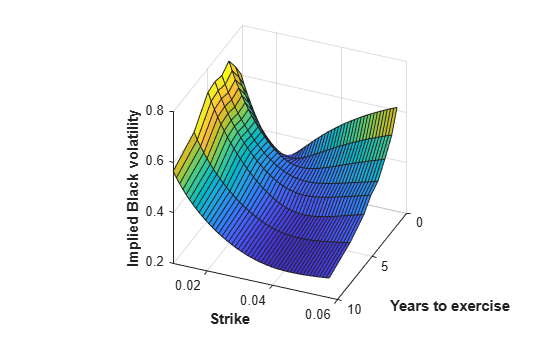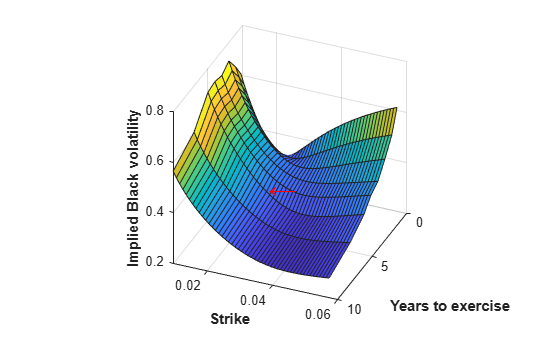# Price a Swaption Using SABR Model and Analytic Pricer

This example shows how to price a swaption using the `SABR` model. First, you construct a swaption volatility surface from market volatilities by calibrating the `SABR` model parameters separately for each swaption maturity using the `SABR` analytic pricer. You then compute the swaption price by using the implied Black volatility on the surface with the `SABR` analytic pricer.

### Step 1. Load market swaption volatility data.

Load the zero curve and market implied Black volatility data for swaptions.

```Settle = datetime(2013, 6, 14); ZeroDates = Settle + [calmonths([1 3 6]) calyears([1 2 3 4 5 6 7 8 9 10 12 15 20])]'; ZeroRates = [0.22 0.31 0.45 0.73 0.54 0.72 1.22 1.54 1.83 1.92 ... 2.16 2.32 2.52 2.93 3.12 3.36]'/100; ZeroCurve = ratecurve("zero",Settle,ZeroDates,ZeroRates,'Compounding',1); ExerciseDates = Settle + [calmonths(3) calyears([1 2 3 4 5 7 10])]'; YearsToExercise = yearfrac(Settle,ExerciseDates,1); NumMaturities = length(YearsToExercise); MarketVolatilities = [ ... 56.5 52.7 49.1 44.9 43.5 40.5 34.8 32.2 45.8 46.2 44.2 41.1 39.1 36.1 33.2 31.3 34.7 38.8 39.0 37.2 36.8 33.2 30.1 28.1 33.9 35.9 36.9 35.8 34.2 30.5 29.0 27.0 40.8 41.2 38.6 37.0 35.3 32.0 29.5 26.5 45.1 42.8 41.2 38.3 37.2 33.2 30.3 27.2 50.2 45.4 43.2 39.9 38.0 34.1 31.5 28.3]/100; MarketStrikes = [ ... 1.02 1.31 1.78 2.08 2.21 2.34 2.60 2.69; 1.52 1.81 2.28 2.58 2.71 2.84 3.10 3.19; 2.02 2.31 2.78 3.08 3.21 3.34 3.60 3.69; 2.52 2.81 3.28 3.58 3.71 3.84 4.10 4.19; 3.02 3.31 3.78 4.08 4.21 4.34 4.60 4.69; 3.52 3.81 4.28 4.58 4.71 4.84 5.10 5.19; 4.02 4.31 4.78 5.08 5.21 5.34 5.60 5.69]/100; CurrentForwardValues = MarketStrikes(4,:)```
```CurrentForwardValues = 1×8 0.0252 0.0281 0.0328 0.0358 0.0371 0.0384 0.0410 0.0419 ```
`ATMVolatilities = MarketVolatilities(4,:)`
```ATMVolatilities = 1×8 0.3390 0.3590 0.3690 0.3580 0.3420 0.3050 0.2900 0.2700 ```

The current underlying forward rates and the corresponding at-the-money volatilities across the eight swaption maturities are represented in the fourth rows of the two matrices.

### Step 2. Calibrate the SABR model parameters for each swaption maturity.

When you use a static `SABR` model, where the model parameters are assumed to be constant with respect to time, the parameters are calibrated separately for each swaption maturity (years to exercise) in a `for` loop using the `SABR` analytic pricer. To better represent market at-the-money volatilities, the `Alpha` parameter values are implied by the market at-the-money volatilities (for details, see Method 2 in Calibrate SABR Model Using Analytic Pricer).

```% Define the predetermined Beta, calibrate SABR model parameters for each % swaption maturity, and display the calibrated parameters in a table. Beta = 0.5; Betas = repmat(Beta, NumMaturities, 1); Alphas = zeros(NumMaturities, 1); Rhos = zeros(NumMaturities, 1); Nus = zeros(NumMaturities, 1); options = optimoptions('lsqnonlin','Display','none'); for k = 1:NumMaturities % This function solves the SABR at-the-money volatility equation as a % polynomial of Alpha. alpharoots = @(Rho,Nu) roots([... (1 - Beta)^2*YearsToExercise(k)/24/CurrentForwardValues(k)^(2 - 2*Beta) ... Rho*Beta*Nu*YearsToExercise(k)/4/CurrentForwardValues(k)^(1 - Beta) ... (1 + (2 - 3*Rho^2)*Nu^2*YearsToExercise(k)/24) ... -ATMVolatilities(k)*CurrentForwardValues(k)^(1 - Beta)]); % This function converts at-the-money volatility into Alpha by picking the % smallest positive real root. atmVol2SabrAlpha = @(Rho,Nu) min(real(arrayfun(@(x) ... x*(x>0) + realmax*(x<0 || abs(imag(x))>1e-6), alpharoots(Rho,Nu)))); % Fit Rho and Nu (while converting at-the-money volatility into Alpha). objFun = @(X) MarketVolatilities(:,k) - ... volatilities(finpricer("Analytic", 'Model', finmodel("SABR", ... 'Alpha', atmVol2SabrAlpha(X(1), X(2)), 'Beta', Beta, 'Rho', X(1), 'Nu', X(2)), ... 'DiscountCurve', ZeroCurve), ExerciseDates(k), CurrentForwardValues(k), MarketStrikes(:,k)); X = lsqnonlin(objFun, [0 0.5], [-1 0], [1 Inf], options); Rho = X(1); Nu = X(2); % Get final Alpha from the calibrated parameters. Alpha = atmVol2SabrAlpha(Rho, Nu); Alphas(k) = Alpha; Rhos(k) = Rho; Nus(k) = Nu; end CalibratedParameters = array2table([Alphas Betas Rhos Nus],... 'VariableNames',{'Alpha' 'Beta' 'Rho' 'Nu'},... 'RowNames',{'3M into 10Y';'1Y into 10Y';... '2Y into 10Y';'3Y into 10Y';'4Y into 10Y';... '5Y into 10Y';'7Y into 10Y';'10Y into 10Y'})```
```CalibratedParameters=8×4 table Alpha Beta Rho Nu ________ ____ ________ _______ 3M into 10Y 0.051895 0.5 0.40869 1.4054 1Y into 10Y 0.054381 0.5 0.28066 1.1234 2Y into 10Y 0.057325 0.5 0.2166 0.97407 3Y into 10Y 0.057243 0.5 0.19837 0.82932 4Y into 10Y 0.053387 0.5 0.17304 0.81441 5Y into 10Y 0.04673 0.5 0.11618 0.80138 7Y into 10Y 0.046986 0.5 0.1554 0.63377 10Y into 10Y 0.04443 0.5 0.080169 0.52515 ```

### Step 3. Construct a volatility surface.

Use the calibrated `SABR` model to compute new volatilities at any strike value to produce a smooth smile for a given maturity. This can be repeated for each maturity to form a volatility surface.

Compute volatilities using the calibrated models for each maturity and plot the volatility surface.

```PlottingStrikes = (0.95:0.1:5.8)'/100; ComputedVols = zeros(length(PlottingStrikes), NumMaturities); for k = 1:NumMaturities SABRModel = finmodel("SABR", ... 'Alpha', Alphas(k), 'Beta', Betas(k), 'Rho', Rhos(k), 'Nu', Nus(k)); ComputedVols(:,k) = volatilities(finpricer("Analytic", ... 'Model', SABRModel, 'DiscountCurve', ZeroCurve), ... ExerciseDates(k), CurrentForwardValues(k), PlottingStrikes); end figure; surf(YearsToExercise, PlottingStrikes, ComputedVols); xlim([0 10]); ylim([0.0095 0.06]); zlim([0.2 0.8]); view(113,32); set(gca, 'Position', [0.13 0.11 0.775 0.815], ... 'PlotBoxAspectRatioMode', 'manual'); xlabel('Years to exercise', 'Fontweight', 'bold'); ylabel('Strike', 'Fontweight', 'bold'); zlabel('Implied Black volatility', 'Fontweight', 'bold');```Note that in this volatility surface, the smiles tend to get flatter for longer swaption maturities (years to exercise). This is consistent with the Nu parameter values tending to decrease with swaption maturity, as shown in the table for `CalibratedParameters`.

### Step 4. Use the `SABR` analytic pricer to price a swaption.

Use the `SABR` analytic pricer to price a swaption that matures in five years. First, create the underlying 10-year swap instrument starting in five years and create the `Swaption` instrument for this underlying `Swap`. Then create the `SABR` model for this swaption maturing in five years, which is used for creating the `SABR` analytic pricer.

```% Create the underlying 10-year swap starting in 5 years. MaturityIdx = 6; SwapStartDate = ExerciseDates(MaturityIdx); SwapMaturity = SwapStartDate + calyears(10); Swap = fininstrument("Swap", 'Maturity', SwapMaturity, ... 'LegRate', [0 0], "LegType",["fixed" "float"],... "ProjectionCurve", ZeroCurve, "StartDate", SwapStartDate)```
```Swap = Swap with properties: LegRate: [0 0] LegType: ["fixed" "float"] Reset: [2 2] Basis: [0 0] Notional: 100 LatestFloatingRate: [NaN NaN] ResetOffset: [0 0] DaycountAdjustedCashFlow: [0 0] ProjectionCurve: [1x2 ratecurve] BusinessDayConvention: ["actual" "actual"] Holidays: NaT EndMonthRule: [1 1] StartDate: 14-Jun-2018 Maturity: 14-Jun-2028 Name: "" ```
```% Create the swaption (for the underlying 10-year swap) maturing in five % years. SwaptionExerciseDate = SwapStartDate; Reset = 1; OptSpec = 'call'; Strike = 0.0272; Swaption = fininstrument("Swaption", 'Strike', Strike, ... 'ExerciseDate', SwaptionExerciseDate, 'Swap', Swap, 'OptionType', OptSpec)```
```Swaption = Swaption with properties: OptionType: "call" ExerciseStyle: "european" ExerciseDate: 14-Jun-2018 Strike: 0.0272 Swap: [1x1 fininstrument.Swap] Name: "" ```
```% Create the SABR model for the swaption maturing in five years. SABRModel = finmodel("SABR", ... 'Alpha', Alphas(MaturityIdx), 'Beta', Betas(MaturityIdx), ... 'Rho', Rhos(MaturityIdx), 'Nu', Nus(MaturityIdx))```
```SABRModel = SABR with properties: Alpha: 0.0467 Beta: 0.5000 Rho: 0.1162 Nu: 0.8014 Shift: 0 VolatilityType: "black" ```
```% Create the SABR analytic pricer. SABRPricer = finpricer("Analytic", 'Model', SABRModel, 'DiscountCurve', ZeroCurve)```
```SABRPricer = SABR with properties: DiscountCurve: [1x1 ratecurve] Model: [1x1 finmodel.SABR] ```

To visualize the SABR implied Black volatility used in pricing the swaption, first compute the current forward swap rate by using the `parswaprate` function.

`CurrentForwardSwapRate = parswaprate(Swap,ZeroCurve)`
```CurrentForwardSwapRate = 0.0384 ```

Next, compute the SABR implied Black volatility for this `Swaption` by using the `volatilities` function, and it is marked with a red arrow in the figure at the bottom.

```SABRBlackVolatility = volatilities(SABRPricer, ... SwaptionExerciseDate, CurrentForwardSwapRate, Strike)```
```SABRBlackVolatility = 0.3665 ```
```text (YearsToExercise(MaturityIdx), Strike, SABRBlackVolatility, ... '\leftarrow', 'Color', 'r', 'FontWeight', 'bold', 'FontSize', 22);```Finally, price the swaption using the `price` function of the `SABR` analytic pricer.

`SwaptionPrice = price(SABRPricer, Swaption)`
```SwaptionPrice = 13.0141 ```

### References

 Hagan, Patrick S., Deep Kumar, Andrew S. Lesniewski, and Diana E. Woodward. "Managing Smile Risk." Wilmott Magazine. (January 2002): 84–108.

 West, Graeme. "Calibration of the SABR Model in Illiquid Markets." Applied Mathematical Finance. 12, no. 4 (December 2005): 371–385.